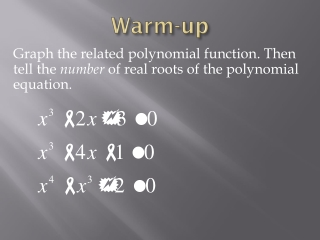Download PresentationWarm-up

Loading in 2 Seconds...

# Warm-up

Download Presentation## Warm-up

- - - - - - - - - - - - - - - - - - - - - - - - - - - E N D - - - - - - - - - - - - - - - - - - - - - - - - - - -
##### Presentation Transcript

1. Warm-up Graph the related polynomial function. Then tell the number of real roots of the polynomial equation.

2. One root.

3. Three roots

4. No roots

5. 2.5 Using Technology to Approximate Roots of Polynomial Equations • x-intercepts • y-intercept • Local minimum • Local maximum

6. 2.5 Using Technology to Approximate Roots of Polynomial Equations Calculator

7. 2.5 Using Technology to Approximate Roots of Polynomial Equations Finding zeros on a table. Root Change of Sign

8. 2.5 Using Technology to Approximate Roots of Polynomial Equations Finding zeros on a table. Better Approximations

9. 2.5 Using Technology to Approximate Roots of Polynomial Equations there exists

10. Section 2.5 Worksheet #1-4 Page 78 #7,9,11,15

11. Quiz

12. Make a sketch and write an equation for each situation: 1. A picture has a height that is 4/3 its width. It is to be enlarged to have an area of 192 square inches. What will be the dimensions of the enlargement? 2. A garden measuring 12 meters by 16 meters is to have a pedestrian pathway installed all around it, increasing the total area to 285 square meters. What will be the width of the pathway? 3. You have to make a square-bottomed, unlidded box with a height of three inches and a volume of approximately 42 cubic inches. You will be taking a piece of cardboard, cutting three-inch squares from each corner, scoring between the corners, and folding up the edges. What should be the dimensions of the cardboard, to the nearest quarter inch?

13. Let "w" stand for the width of the picture. The height h is 4/3 the width, so h = (4/3)w. Then the area is A = hw = [(4/3)w][w] = (4/3)w2 = 192. I need to solve this "area" equation for the value of the width, and then back-solve to find the value of the height. (4/3)w2 = 192 w2 = 144 w = ± 12